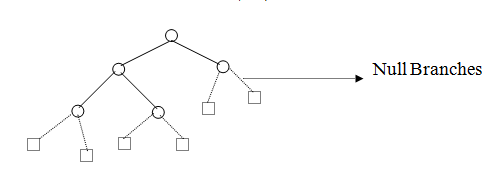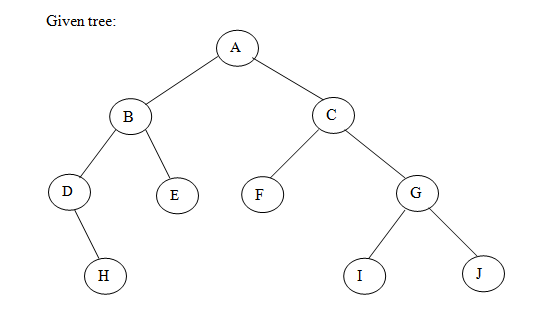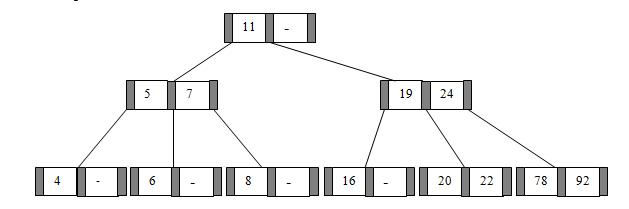## Print this Job Post# Data Structure Aptitude

1. What is data structure?
A data structure is a way of organizing data that considers not only the items stored, but also their relationship to each other. Advance knowledge about the relationship between data items allows designing of efficient algorithms for the manipulation of data.

2. List out the areas in which data structures are applied extensively?
Compiler Design,
Operating System,
Database Management System,
Statistical analysis package,
Numerical Analysis,
Graphics,
Artificial Intelligence,
Simulation

3. What are the major data structures used in the following areas : RDBMS, Network data model & Hierarchical data model.
RDBMS                         – Array  (i.e. Array of structures)
Network data model      – Graph
Hierarchical data model – Trees

4. If you are using C language to implement the heterogeneous linked list, what pointer type will you use?
The heterogeneous linked list contains different data types in its nodes and we need a link, pointer to connect them. It is not possible to use ordinary pointers for this. So we go for void pointer. Void pointer is capable of storing pointer to any type as it is a generic pointer type.

5. Minimum number of queues needed to implement the priority queue?
Two. One queue is used for actual storing of data and another for storing priorities.

6. What is the data structures used to perform recursion?
Stack. Because of its LIFO (Last In First Out) property it remembers its ‘caller’ so knows whom to return when the function has to return. Recursion makes use of system stack for storing the return addresses of the function calls.
Every recursive function has its equivalent iterative (non-recursive) function. Even when such equivalent iterative procedures are written, explicit stack is to be used.

7. What are the notations used in Evaluation of Arithmetic Expressions using prefix and postfix forms?
Polish and Reverse Polish notations.

8. Convert the expression ((A + B) * C – (D – E) ^ (F + G)) to equivalent Prefix and Postfix notations.
Prefix Notation:
^ - * +ABC - DE + FG
Postfix Notation:
AB + C * DE - - FG + ^

9. Sorting is not possible by using which of the following methods?
(a) Insertion
(b) Selection
(c) Exchange
(d) Deletion
(d) Deletion.
Using insertion we can perform insertion sort, using selection we can perform selection sort, using exchange we can perform the bubble sort (and other similar sorting methods). But no sorting method can be done just using deletion.

10. A binary tree with 20 nodes has_______null branches?
21
Let us take a tree with 5 nodes (n=5)It will have only 6 (i.e., 5+1) null branches. In general, A binary tree with n nodes has exactly n+1null nodes.

11. What are the methods available in storing sequential files?
Straight merging,
Natural merging,
Polyphase sort,
Distribution of Initial runs.

12. How many different trees are possible with 10 nodes ?
1014
For example, consider a tree with 3 nodes (n=3), it will have the maximum combination of 5 different (i.e., 23 - 3 = 5) trees.In general:
If there are n nodes, there exist 2n-n different trees.

13. List out few of the Application of tree data-structure?
The manipulation of Arithmetic expression,
Symbol Table construction,
Syntax analysis.

14. List out few of the applications that make use of Multilinked Structures?
Sparse matrix,
Index generation.

15. In tree construction which is the suitable efficient data structure?
(a) Array
(c) Stack
(d) Queue
(e) none

16. What is the type of the algorithm used in solving the 8 Queens problem?
Backtracking

17. In an AVL tree, at what condition the balancing is to be done?
If the ‘pivotal value’ (or the ‘Height factor’) is greater than 1 or less than –1.

18. What is the bucket size, when the overlapping and collision occur at same time?
One. If there is only one entry possible in the bucket, when the collision occurs, there is no way to accommodate the colliding value. This results in the overlapping of values.

19. Traverse the given tree using Inorder, Preorder and Postorder traversals.Inorder : D H B E A F C I G J
Preorder: A B D H E C F G I J
Postorder: H D E B F I J G C A

20. There are 8, 15, 13, 14 nodes were there in 4 different trees. Which of them could have formed a full binary tree?
15.
In general:
There are 2n-1 nodes in a full binary tree.
By the method of elimination:
Full binary trees contain odd number of nodes. So there cannot be full binary trees with 8 or 14 nodes, so rejected. With 13 nodes you can form a complete binary tree but not a full binary tree. So the correct answer is 15.
*Note:
Full and Complete binary trees are different. All full binary trees are complete binary trees but not vice versa.

21. In the given binary tree, using array you can store the node 4 at which location?At location  6

22. Sort the given values using Quick Sort?

65 70 75 80 85 60 55 50 45
Sorting takes place from the pivot value, which is the first value of the given elements, this is marked bold. The values at the left pointer and right pointer are indicated using L and R respectively.

65 70L 75 80 85 60 55 50 45R

Since pivot is not yet changed the same process is continued after interchanging the values at L and R positions

65 45 75L 80 85 60 55 50R 70

65 45 50 80L 85 60 55R 75 70

65 45 50 55 85L 60R 80 75 70

65 45 50 55 60R 85L 80 75 70

When the L and R pointers cross each other the pivot value is interchanged with the value at right pointer. If the pivot is changed it means that the pivot has occupied its original position in the sorted order (shown in bold italics) and hence two different arrays are formed, one from start of the original array to the pivot position-1 and the other from pivot position+1 to end.

60L 45 50 55R 65 85L 80 75 70R

55L 45 50R 60 65 70R 80L 75 85

50L 45R 55 60 65 70 80L  75R 85

In the next pass we get the sorted form of the array.

45 50 55 60 65 70 75 80 85

23. For the given graph, draw the DFS and BFS?BFS:  A X G H P E M Y J

DFS: A X H P E Y M J G

24. Classify the Hashing Functions based on the various methods by which the key value is found.
Direct method,
Subtraction method,
Modulo-Division method,
Digit-Extraction method,
Mid-Square method,
Folding method,
Pseudo-random method.

25. What are the types of Collision Resolution Techniques and the methods used in each of the type?
The methods used include:
Overflow block,
The methods used include:
Binary tree…

26. In RDBMS, what is the efficient data structure used in the internal storage representation?
B+ tree. Because in B+ tree, all the data is stored only in leaf nodes, that makes searching easier. This corresponds to the records that shall be stored in leaf nodes.

27. Draw the B-tree of order 3 created by inserting the following data arriving in sequence –
92  24  6  7  11  8  22  4  5  16  19  20  7828. Of the following tree structure, which is, efficient considering space and time complexities?
(a) Incomplete Binary Tree
(b) Complete Binary Tree
(c) Full Binary Tree
(b) Complete Binary Tree.
By the method of elimination:
Full binary tree loses its nature when operations of insertions and deletions are done. For incomplete binary trees, extra storage is required and overhead of NULL node checking takes place. So complete binary tree is the better one since the property of complete binary tree is maintained even after operations like additions and deletions are done on it.

29. What is a spanning Tree?
A spanning tree is a tree associated with a network. All the nodes of the graph appear on the tree once. A minimum spanning tree is a spanning tree organized so that the total edge weight between nodes is minimized.

30. Does the minimum spanning tree of a graph give the shortest distance between any 2 specified nodes?
No.
Minimal spanning tree assures that the total weight of the tree is kept at its minimum. But it doesn’t mean that the distance between any two nodes involved in the minimum-spanning tree is minimum.

31. Convert the given graph with weighted edges to minimal spanning tree.the equivalent minimal spanning tree is:32. Which is the simplest file structure?
(a) Sequential
(b) Indexed
(c) Random
(a) Sequential

33. Whether Linked List is linear or Non-linear data structure?

• According to Access strategies Linked list is a linear one.
• According to Storage Linked List is a Non-linear one.

34. Draw a binary Tree for the expression :

A * B - (C + D) * (P / Q)35. For the following COBOL code, draw the Binary tree?
01 STUDENT_REC.
02 NAME.
03 FIRST_NAME PIC X(10).
03 LAST_NAME PIC X(10).

02 YEAR_OF_STUDY.
03 FIRST_SEM PIC XX.
03 SECOND_SEM PIC XX.